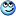# Identify 2 Unknown Elements in a Chemical Equation?

Lori

## Homework Statement

A silly sample of a metallic element M, reacts completely with 0.0237 mols of X2 to form 6.78 g MX. What are the identities of M and X?

## Homework Equations

mols = grams/molar mass[/B]

## The Attempt at a Solution

(kinda have no clue what to do because i'm not sure what to do. There aren't any elements to compare the unknown with !)

Here's the equation i got

M + X2 > MX
balancing it: 2M + X2 > 2MX

6.78g MX * (???)

I honestly don't know what to start with and would appreciate if someone gave me hints ! :(

DoItForYourself
Hello,

As long as the reaction is complete and you know the moles of one of the substances, you automatically know the moles of the other substances.

The mass of one of them is given, so you can calculate its molar mass. This can lead you to the solution (search in periodic table).

•mfb
Lori
Hello,

As long as the reaction is complete and you know the moles of one of the substances, you automatically know the moles of the other substances.

The mass of one of them is given, so you can calculate its molar mass. This can lead you to the solution (search in periodic table).
I get that MX has a molar mass of 143 g/mol but that's not for separate elements!

Actually, i just figured out that i was given 5.11 grams of X?

Would M be Ag? I found its molar mass to be 107.8g/mol

I also found X to be Ga because i found its molar mass is 70.46 g/mol

(Im pretty sure i messed up! .-. I'll post my work when i wake up~ tomorrow xD)

Last edited by a moderator:
mfb
Mentor
Actually, i just figured out that i was given 5.11 grams of X?
That helps.
Would M be Ag? I found its molar mass to be 107.8g/mol I also found X to be Ga because i found its molar mass is 70.46 g/mol
How did you get these values?

Lori
That helps.How did you get these values?
I was actually given 5.11 grams of M

Lori
0.0237 mols X2 * (2 mol MX / 1 mol X2)
=0.0484 mol MX

6.78g MX/0.0484 mol Mx =143 g/mol

0.0237 mol x2 * (2 mol M/2 mol X) = ?? Mols M

5 .11g M / ?? Mols M = 107.8 g/mol

mfb
Mentor
I was actually given 5.11 grams of M
Okay, that makes more sense.
I agree with silver then.

The molar mass of X2 that you probably calculated earlier is fine as well - what does that imply for an atom of X?

DoItForYourself
0.0237 mols X2 * (2 mol MX / 1 mol X2)
=0.0484 mol MX

6.78g MX/0.0484 mol Mx =143 g/mol

0.0237 mol x2 * (2 mol M/2 mol X) = ?? Mols M

5 .11g M / ?? Mols M = 107.8 g/mol

You calculated the molar mass of MX to be 143 g/moland the molar mass of M to be 107.8 g/mol.
So you can calculate the molar mass of X directly from these data.

Beware: It is 0.0474 and not 0.0484 mols MX.

Borek
Mentor
I also found X to be Ga because i found its molar mass is 70.46 g/mol

Beware: X2.

I like Serena
Homework Helper
Would M be Ag? I found its molar mass to be 107.8g/mol

That must be why the problem statement said: 'A silly sample of a metallic element M.'
silly = silvery•Lori and DoItForYourself
Lori
That must be why the problem statement said: 'A silly sample of a metallic element M.'
silly = silveryActually, i ready 5.11 g as Silly ! XD Look~
https://imgur.com/a/OdEcl•I like Serena
Borek
Mentor
Funny, as 5.11 g is not necessary to solve the problem.

Lori
Since there is 5.11 g of M and 6.78 g of MX in the products, can i subtract the two to get grams of X2?

So i can do 0.0237 mols X2 * (2moles X / 1 mole X2) = 0.0474 moles X

6.78 g - 5.11 g = 1.67 g X2

1.67 g X2 / (.0237 moles X2) = 70.46 g/mols X2 /2 = 35.23 g/mol of X = Chlorine (35.453 g/mol)

•DoItForYourself
Lori
You calculated the molar mass of MX to be 143 g/moland the molar mass of M to be 107.8 g/mol.
So you can calculate the molar mass of X directly from these data.

Beware: It is 0.0474 and not 0.0484 mols MX.
How do you use just the molar masses to figure out X?

DoItForYourself
How do you use just the molar masses to figure out X?

As long as MW(MX)=143 and MW(M)=107.8, you can use the equation MW(MX)=MW(M)+MW(X). The only unknown is MW(X).

From the MW (molecular weight) of X, you can define the chemical element X directly.

•Lori
Lori
As long as MW(MX)=143 and MW(M)=107.8, you can use the equation MW(MX)=MW(M)+MW(X). The only unknown is MW(X).

From the MW (molecular weight) of X, you can define the chemical element X directly.
Oh ! ! that makes sense! Thanks! This confirms my answer too!